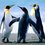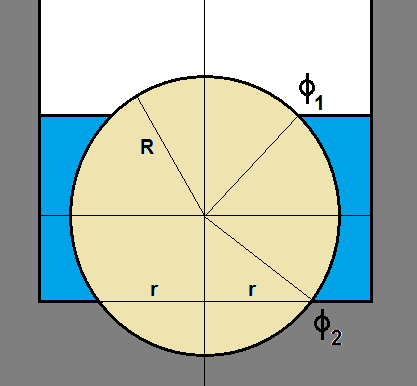# Fluids mechanics

A sphere of radius $R$, is placed in a hole of radius $r$ ($R>r$), at the bottom of a beaker. What is the minimum mass of sphere required for it to not leave the hole for any amount of liquid of density $\rho$ poured into the beaker?Note by Kudo Shinichi
2 years, 3 months ago

This discussion board is a place to discuss our Daily Challenges and the math and science related to those challenges. Explanations are more than just a solution — they should explain the steps and thinking strategies that you used to obtain the solution. Comments should further the discussion of math and science.

When posting on Brilliant:

• Use the emojis to react to an explanation, whether you're congratulating a job well done , or just really confused .
• Ask specific questions about the challenge or the steps in somebody's explanation. Well-posed questions can add a lot to the discussion, but posting "I don't understand!" doesn't help anyone.
• Try to contribute something new to the discussion, whether it is an extension, generalization or other idea related to the challenge.
• Stay on topic — we're all here to learn more about math and science, not to hear about your favorite get-rich-quick scheme or current world events.

MarkdownAppears as
*italics* or _italics_ italics
**bold** or __bold__ bold
- bulleted- list
• bulleted
• list
1. numbered2. list
1. numbered
2. list
Note: you must add a full line of space before and after lists for them to show up correctly
paragraph 1paragraph 2

paragraph 1

paragraph 2

[example link](https://brilliant.org)example link
> This is a quote
This is a quote
    # I indented these lines
# 4 spaces, and now they show
# up as a code block.

print "hello world"
# I indented these lines
# 4 spaces, and now they show
# up as a code block.

print "hello world"
MathAppears as
Remember to wrap math in $$ ... $$ or $ ... $ to ensure proper formatting.
2 \times 3 $2 \times 3$
2^{34} $2^{34}$
a_{i-1} $a_{i-1}$
\frac{2}{3} $\frac{2}{3}$
\sqrt{2} $\sqrt{2}$
\sum_{i=1}^3 $\sum_{i=1}^3$
\sin \theta $\sin \theta$
\boxed{123} $\boxed{123}$

## Comments

Sort by:

Top Newest

@Azimuddin Sheikh I think I will post a problem on thisThe sphere of radius $R$ sits in a hole of radius $r$ at the bottom of a container. Suppose that the center of the sphere is at the origin $(x,y,z) = (0,0,0)$. Let $\phi$ be the angle with respect to the positive $z$ axis, and let $\theta$ be the angle with respect to the positive $x$ axis.

Water fills the container from the bottom to some height. Let $\phi_2$ correspond to the bottom contact point between the water and the sphere, and let $\phi_1$ correspond to the top contact point between the water and the sphere.

The expression for $\phi_2$ in terms of $r$ and $R$ is:

$\phi_2 = \frac{\pi}{2} + acos \Big( \frac{r}{R}\Big )$

The expression for the $z$ coordinate of an arbitrary point on the sphere is:

$z = R \, cos(\phi)$

Here, $\phi = 0$ corresponds to the top of the sphere. The pressure is:

$P = \rho \, g \, R \, [cos \phi_1 - cos \phi]$

The infinitesimal sphere surface area is:

$dA = R^2 \, sin \phi \, d \theta \, d \phi$

The infinitesimal pressure force magnitude is:

$dF = P \, dA = \rho \, g \, R^3 \, sin \phi \, [cos \phi_1 - cos \phi] \, d \theta \, d \phi$

The infinitesimal force in the $z$ direction is:

$dF_z = dF \, \frac{-z}{R} = dF \, (-cos \phi) = -\rho \, g \, R^3 \, sin \phi \, cos \phi \, [cos \phi_1 - cos \phi] \, d \theta \, d \phi$

The total force in the $z$ direction is:

$F_z = -\rho \, g \, R^3 \int_{\phi_1}^{\phi_2} \int_0^{2 \pi} sin \phi \, cos \phi \, [cos \phi_1 - cos \phi] \, d \theta \, d \phi \\ = - 2 \pi \, \rho \, g \, R^3 \int_{\phi_1}^{\phi_2} sin \phi \, cos \phi \, [cos \phi_1 - cos \phi] \, d \phi \\ = -\frac{\pi \, \rho \, g \, R^3}{3} [ cos \phi_1 - cos \phi_2]^2 \, [cos \phi_1 + 2 cos \phi_2]$

As a sanity check, suppose the hole had zero width, and that the water was filled to the top of the sphere. In this case, $\phi_1 = 0$ and $\phi_2 = \pi$. The $F_z$ expression reduces to:

$F_z = -\frac{\pi \, \rho \, g \, R^3}{3} [ 1 + 1]^2 \, [1 - 2] \\ = \frac{4 \pi \, \rho \, g \, R^3}{3} = V_{sphere} \, \rho \, g = \text{weight of displaced water}$

In general, we take $\phi_2$ as a given, and we must find the value of $\phi_1$ which maximizes the upward pressure force. I will leave this as an exercise for the reader, but it turns out that the upward pressure force is maximized when the following condition is satisfied:

$\phi_1 + \phi_2 = \pi$

So the minimum weight of the sphere, to ensure that it covers the hole unconditionally, is:

$W_{sphere-min} = -\frac{\pi \, \rho \, g \, R^3}{3} [ cos \phi_1 - cos \phi_2]^2 \, [cos \phi_1 + 2 cos \phi_2] \\ \phi_2 = \frac{\pi}{2} + acos \Big( \frac{r}{R}\Big ) \\ \phi_1 = \pi - \phi_2 = \frac{\pi}{2} - acos \Big( \frac{r}{R}\Big )$

- 2 years, 3 months ago

Log in to reply

@Steven Chase Sir thx for your method great way .!! . yeah sure post a problem sir.

- 2 years, 3 months ago

Log in to reply

@Steven Chase Sir BTW can't it be much simplified with the help.of conservation of energy ? Without using force and integration method ?? Also sir if u have some time can u pls try this problem also out ?? https://brilliant.org/discussions/thread/classical-mechanics-problem-3/?ref_id=1563236

- 2 years, 3 months ago

Log in to reply

This is a stasis problem, so how would energy conservation be relevant?

- 2 years, 3 months ago

Log in to reply

Oh yes sir nvm was thinking about some other problem . BTW sir may u try this one if u have some time ?https://brilliant.org/discussions/thread/classical-mechanics-problem-3/?ref_id=1563236

- 2 years, 3 months ago

Log in to reply

@steven chase sir pls help ,ideas pls. @ram mohith pls help ?

- 2 years, 3 months ago

Log in to reply

@Steven Chase and @Ram Mohith you may want to check this note.......

- 2 years, 3 months ago

Log in to reply

This is an interesting one. I'll start thinking about it.

- 2 years, 3 months ago

Log in to reply

Good to know about that its interesting 😊

- 2 years, 3 months ago

Log in to reply

How can a sphere of radius $R$ be placed in a hole of radius $r$ if $R >r$. I didn't understand the first part of the question. Is there any diagram.

- 2 years, 3 months ago

Log in to reply

@Ram Mohith bro , in a beaker there is hole in the basement where a sphere of radius R is placed , off course some part of sphere is outside in air and other inside the liquid

- 2 years, 3 months ago

Log in to reply

×

Problem Loading...

Note Loading...

Set Loading...